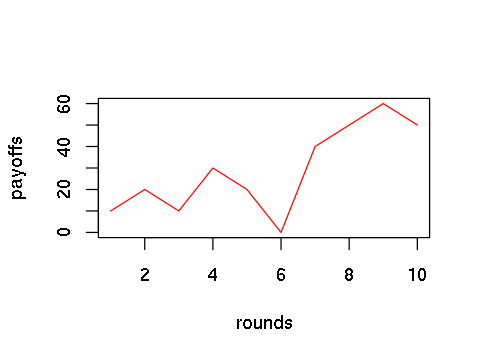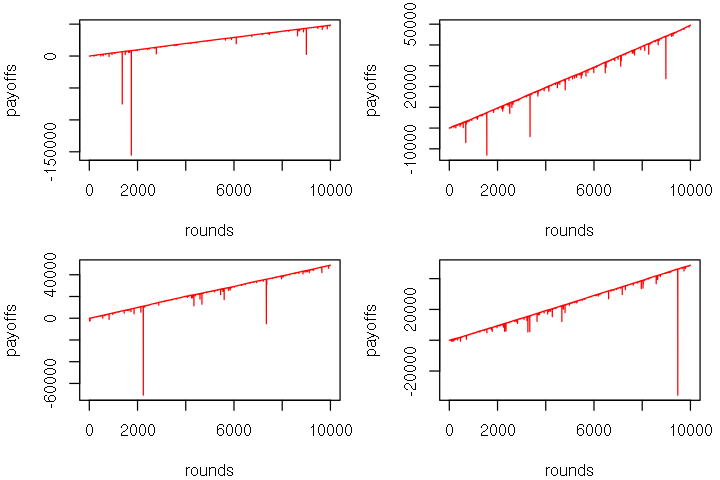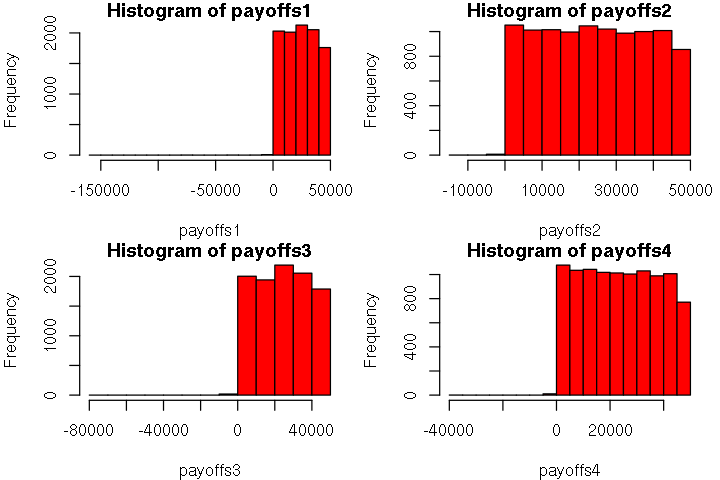# The Game of Craps¶

Oct. 7, 2018 $\newcommand{\bs}{\boldsymbol}$ $\newcommand{\argmin}{\underset{\bs{#1}}{\text{arg min}}\,}$ $\newcommand{\argmax}{\underset{\bs{#1}}{\text{arg max}}\,}$ $\newcommand{\tr}{^{\top}}$ $\newcommand{\norm}{\left|\left|\,#1\,\right|\right|}$ $\newcommand{\given}{\,|\,}$

To have some fun with Monte Carlo simulation in R, let's analyze the game of craps, a popular casino game. We can learn a lot about gambling using Monte Carlo simulation.## Rules¶

During the game of craps, players take turn rolling two dice. The player who thows the dice is called the Shooter. As the shooter, the player must place a bet on either Pass or Don't Pass. All other players can place bets as well, but not mandatory. The shooter will make a Come-out roll, so that two dice must hit the back wall in order to be a valid throw. Any players who didn't bet prior to the Come-out roll are no longer eligible to make "Pass" or "Don't Pass" bet. Instead, they may place a Come or Don't Come bet. The following are the rules.

• If the Come-out rolls is any of $\{4, 5, 6, 8, 9, 10\}$,
• A "Point" (let's call $P_{\text{pass}}$) is established as the rolled number for the players who place "Pass" or "Don't Pass" bets.
• The shooter keeps rolling.
• If the next roll is $P_{\text{pass}}$,
• "Pass" wins.
• "Don't Pass" loses.
• Round ends.
• If the next roll is 7 (called seven-out),
• "Pass" loses.
• "Don't Pass" wins.
• Round ends.
• New players may bet on "Come" or "Don't Come" any time before $P_{\text{pass}}$ or 7 is rolled.
• The new player follow the same rule as the original players with an exceptions.
• The new player's "point" will be the number rolled among $\{4, 5, 6, 8, 9, 10\}$ after the bet, called $P_{\text{come}}$.
• After a point is established, the player who bet on "Pass" or "Come" has the option to place the Taking the Odds bets. The Odds pays 2 to 1 on points of 4 and 10, 3 to 2 on a 5 and 9, and 6 to 5 on a 6 and 8. The player can bet up to three times his "Pass" or "Come" bet on the odds after a point of a 4 or 10, four times after a 5 or 9, and five times after a 6 or 8.
• After a point is established, the player who bet on "Don't Pass" or "Don't Come" has the option to place the Laying the Odds bets. The Odds pays 1 to 2 against points of 4 and 10, 2 to 3 against a 5 and 9, and 5 to 6 against a 6 and 8. The player can bet up to six times his "Don't Pass" or "Don't Come" bet for any point.
• If the come-out roll is 2, 3 or 12 (called "craps"),
• "Pass" loses.
• "Don't Pass" wins if the roll is 2 or 3, and ties if the roll is 12.
• Round ends. (Shooter may continue to make "Come-out" rolls during the next round.)
• If the come-out roll is 7 or 11 (called "natural"),
• "Pass" wins.
• "Don't Pass" loses
• Round ends.

There are several other betting options out there, but those result in a very high house edge. For simplicity we only consider Pass Line bets and Taking the Odds bets.

## Pass Line Bets¶

Finding the exact house edge for a simple strategy is not bad, but for more complicated strategies, the computation becomes tedious. Instead, we can use Monte Carlo simulation to get a quick estimate of the probability. First we define a function that outputs the sum of two dice.

In :
roll <- function() {
sum(sample(1:6, 2, replace=T))
}


Suppose a point is established, we define a function that computes the outcome recursively.

In :
craps.continue <- function(point) {
#     returns 1 if a point is rolled before seven-out
#     returns -1 if seven-out
roll <- roll()
if (roll==7){
return(-1)
} else if (roll==point) {
return(1)
} else {
craps.continue(point)
}
}


Now we are ready to define the game craps.pass, where we assume that the Shooter will bet on "Pass" initially and do not do use any other betting until the game ends.

In :
craps.pass <- function(bet=10) {
#     returns payoff of one round on "Pass" bets
payoff <- 0
come_out <- roll()

if (come_out %in% c(2, 3, 12)) {
payoff <- payoff - bet
} else if (come_out %in% c(7, 11)) {
payoff <- payoff + bet
} else {
result <- craps.continue(come_out)
payoff <- payoff + bet*result
}
return(payoff)
}


To approximate the house edge, let's simulate this game a large number of times.

In :
set.seed(1)
rounds <- 1000000

payoff <- rep(0, rounds)
for (i in 1:rounds){
payoff[i] <- craps.pass()
}

mean(payoff)/10

-0.014652

The house edge is around 1.47%, which is pretty close to the real value of 1.41%.

## Martingale Betting Strategy¶

Just for fun, consider the following martingale strategy.

Your initial bet will be 10 dollars. The return on any bet will be the amount that you bet; e.g., if you bet 10 dollars and win, then you win 10 dollars. If you lose the previous game, your next bet requires you to “double up,” that is, if on one game you bet X dollars and win, on the next game you have to bet 2X dollars. If you win on the previous game, your next bet will be 10 dollars.

The idea behind this strategy is that if we lose and double up the bet, surely the next win will cover up all previous losses, plus winning a profit equal to the previous stake. We can write a Monte Carlo simulation as follows.

In :
craps.martingale <- function(rounds=10, verbose=TRUE) {
bet <- 10
payoff <- 0
payoffs <- rep(0, rounds)

for (round in 1:rounds) {
payoff.update <- craps.pass(bet=bet)
payoff <- payoff +  payoff.update
payoffs[round] <- payoff

if (payoff.update < 0){
bet <- 2*bet
} else {
bet <- 10
}

if (verbose==TRUE & payoff.update < 0) {
print(paste("Nay, I lost! New bet:", bet))
} else if (verbose==TRUE & payoff.update > 0) {
print(paste("Yay, I won! New bet:", bet))
}
}
plot(payoffs, type='l', xlab="rounds", col='red')
return(payoffs)
}

In :
set.seed(1)
options(repr.plot.width=4, repr.plot.height=3)
payoffs <- craps.martingale()
print(paste("Total payoff:", payoffs))

 "Yay, I won! New bet: 10"
 "Yay, I won! New bet: 10"
 "Nay, I lost! New bet: 20"
 "Yay, I won! New bet: 10"
 "Nay, I lost! New bet: 20"
 "Nay, I lost! New bet: 40"
 "Yay, I won! New bet: 10"
 "Yay, I won! New bet: 10"
 "Yay, I won! New bet: 10"
 "Nay, I lost! New bet: 20"
 "Total payoff: 50"Let's simulate 4 different games where each game consists of 10000 rounds of using the martingale betting strategy.

In :
options(repr.plot.width=6, repr.plot.height=4)
par(mfrow=c(2,2), mar = c(4, 4, 1, 1))

set.seed(1)
payoffs1 <- craps.martingale(rounds=10000, verbose=FALSE)
payoffs2 <- craps.martingale(rounds=10000, verbose=FALSE)
payoffs3 <- craps.martingale(rounds=10000, verbose=FALSE)
payoffs4 <- craps.martingale(rounds=10000, verbose=FALSE)In :
options(repr.plot.width=6, repr.plot.height=4)
par(mfrow=c(2,2), mar = c(4, 4, 1, 1))

set.seed(1)
hist(payoffs1, col='red')
hist(payoffs2, col='red')
hist(payoffs3, col='red')
hist(payoffs4, col='red')Interesting we see that all betting strategies result in a positive payoff. Is this magic? Certainly not. The biggest downside of the martingale betting strategy is the huge volatility. A player cannot have infinite wealth, so in the long run, the probability that the player go broke is equal to 1! The chance that multiple losses appear in a row in a long series of betting is more common than one expects.

### Optimizing betting strategy¶

Now we consider the "Odds" strategy. Recall:

After a point is established, the player who bet on "Pass" or "Come" has the option to place the Taking the Odds bets. The Odds pays 2 to 1 on points of 4 and 10, 3 to 2 on a 5 and 9, and 6 to 5 on a 6 and 8. The player can bet up to three times his "Pass" or "Come" bet on the odds after a point of a 4 or 10, four times after a 5 or 9, and five times after a 6 or 8.

Let $R$ denote the outcome of each roll. We have $R=2,3,...,12$, with corresponding probabilities

$$P(R=r) = \frac{-|r-7|+6}{36}. \tag{1}$$

This can be obtained simply by counting and note that the probability is maximized at $r=7$.

The odds betting has 0 house edge. To see that suppose the point established is 4. There are 3 ways to win by rolling a 4 next, and 6 ways to lose by rolling a 7. Hence the odds of winning is exactly 1:2. Hence the payoff of 2:1 is fair.

Thus, the optimal strategy that yields the lowest house edge is to bet maximum amount of "Odds" whenever possible. Let's modify our algorithm to include the "Odds" betting.

In :
# Define a new function with odds betting
craps.continue.odds <- function(point) {
roll <- roll()

if (point %in% c(4, 10)) {
if (roll==7){
return(-3)
} else if (roll==point) {
return(6)
} else {
craps.continue.odds(point)
}
} else if (point %in% c(5, 9)) {
if (roll==7){
return(-4)
} else if (roll==point) {
return(6)
} else {
craps.continue.odds(point)
}
} else if (point %in% c(6, 8)) {
if (roll==7){
return(-5)
} else if (roll==point) {
return(6)
} else {
craps.continue.odds(point)
}
}
}

In :
# Updating the craps.pass by including additional payoff from odds betting
craps.pass.odds <- function(bet=10) {
payoff <- 0
come_out <- roll()

if (come_out %in% c(2, 3, 12)) {
payoff <- payoff - bet
} else if (come_out %in% c(7, 11)) {
payoff <- payoff + bet
} else {
result1 <- craps.continue(come_out)
result2 <- craps.continue.odds(come_out)
payoff <- payoff + bet*(result1+result2)
}
return(payoff)
}

In :
# Find expected payoff
set.seed(1)
rounds <- 1000000

payoff <- rep(0, rounds)
for (i in 1:rounds){
payoff[i] <- craps.pass.odds()
}

mean(payoff)/10

-0.011778

Indeed the house edge has decreased slightly!